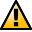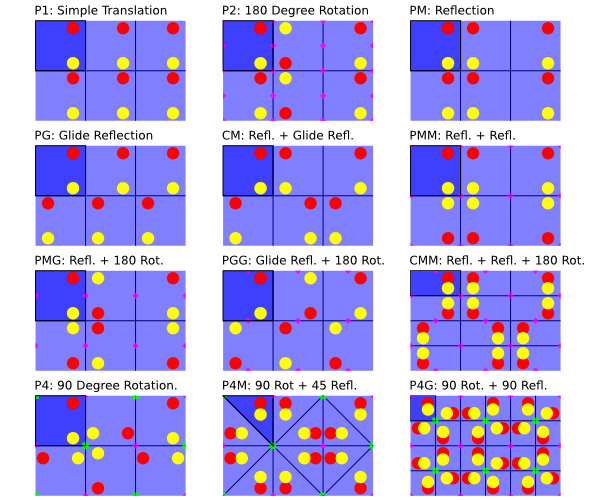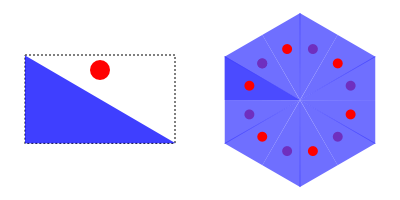WarningThis section not yet updated for Inkscape v0.92.

## Symmetry Tab

The Symmetry tab is at the heart of the tiling process. Understanding the different symmetries is necessary to have full control over the outcome of a tiling. The symmetry of the tiling is selected from the pull-down menu under the Symmetry tab (see previous figure).

There are three regular geometric shapes that can be replicated to cover a surface completely (without gaps or overlaps). These shapes are: triangles, rectangles (parallelograms), and hexagons. A complete set of tiling symmetries requires taking these shapes and adding rotations and reflections. It is known that there are 17 such tiling symmetries. (See: Wikipedia entry.) All 17 symmetries are included in the Inkscape Create Tiled Clones dialog. The symmetries are shown next.Tilings based on a rectangle tile (or 45-45-90 degree triangle). The outlined dark blue tile is the basic unit. Red and yellow dots show the reflection and rotation symmetries. Points of twofold and fourfold rotational symmetry are shown by pink diamonds and green squares, respectively. The P1 and P2 symmetries also work with parallelograms.Tiling based on regular subdivisions of a hexagonal. The outlined dark blue tile is the basic unit. All tilings have points of threefold rotational symmetry (orange triangles). Two also have twofold and sixfold rotational symmetries (pink diamonds and purple hexagons). The pairs of numbers indicate the row and column numbers.

The basic tile for each of the 17 symmetries is shown in dark blue in the preceding figures. Inkscape uses the bounding box of an object to determine the basic tile size. For rectangular base tiles, the bounding box corresponds to the base tile. However, for triangular base tiles, the base tile covers only part of the bounding box area. This can result in tiles overlapping if an object extends outside the base tile shape (but is still within the bounding box) as in the tiling in the introduction to this chapter. Overlapping can also occur if the base tile is altered after the tiles are positioned.On the left is a triangle and circle that are grouped together. The triangle corresponds to the base tile for a P6M symmetry. Note that the red circle is outside the base triangle but is still within the bounding box of the group (and triangle). On the right is a P6M tiling with the triangle and circle. Note how the red circle ends up above some but below other triangles as determined by the order in which the tiling is made.

Inkscape always uses the Geometric bounding box to determine the tile size. This avoids problems when creating a triangular tile with a Stroke where the Visual bounding box doesn't have the same width to height ratio as the Geometric bounding box.

If you need to adjust the base tile size after having created a tiling, you can use the XML Editor dialog to change the parameters inkscape:tile-h and inkscape:tile-w (these will appear after you have cloned the object and are used only if the Use saved size and position of the tile button is checked).